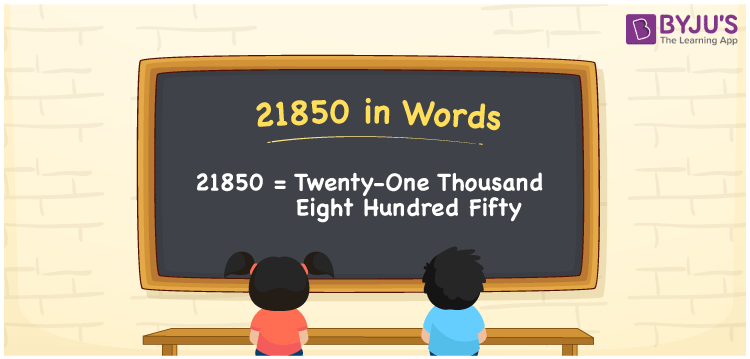# 21850 in Words

21850 in words is written as Twenty-one thousand eight hundred fifty. In both the International System of Numerals and the Indian System of Numerals, 21850 is written as Twenty-one thousand eight hundred fifty. The number 21850 is a Cardinal Number as it could represent some quantity. For example, “the course fee is 21850 rupees”.

 21850 in Words Twenty-one thousand eight hundred fifty Twenty-one thousand eight hundred fifty in Number 21850

## 21850 in English Words

21850 in English words is read as “Twenty-one thousand eight hundred fifty”.## How to Write 21850 in Words?

To write 21850 in words, we shall use the place value chart. In the place value chart, put 2 in the ten thousand, 1 in the thousands, 8 in the hundreds, 5 in the tens and 0 in the ones. Let us make a place value chart to write the number 21850 in words.

 Ten Thousands Thousands Hundreds Tens Ones 2 1 8 5 0

Thus, we can write the expanded form as

2 × Ten Thousand + 1 × Thousand + 8 × Hundred + 5 × Ten + 0 × One

= 2 × 10000 + 1 × 1000 + 8 × 100 + 5 × 10 + 0 × 1

= 20000 + 1000 + 800 +50 + 0

= 21850

= Twenty-one thousand eight hundred fifty.

21850 is a natural number, the successor of 21849 and the predecessor of 21851.

21850 in words – Twenty-one thousand eight hundred fifty

• Is 21850 an odd number? – No
• Is 21850 an even number? – Yes
• Is 21850 a perfect square number? – No
• Is 21850 a perfect cube number? – No
• Is 21850 a prime number? – No
• Is 21850 a composite number? – Yes

## Frequently Asked Questions on 21850 in Words

Q1

### How to write 21850 in words?

21850 in words is written as Twenty-one thousand eight hundred fifty.
Q2

### How to write 21850 in the International and Indian System of Numerals?

In both, the system of numerals, 21850 in words, is written as Twenty-one thousand eight hundred fifty.
Q3

### How to write 21850 in a place value chart?

In the place value chart, write 2 in the ten thousand, 1 in the thousands, 8 in the hundreds, 5 in the tens and 0 in the ones, respectively.